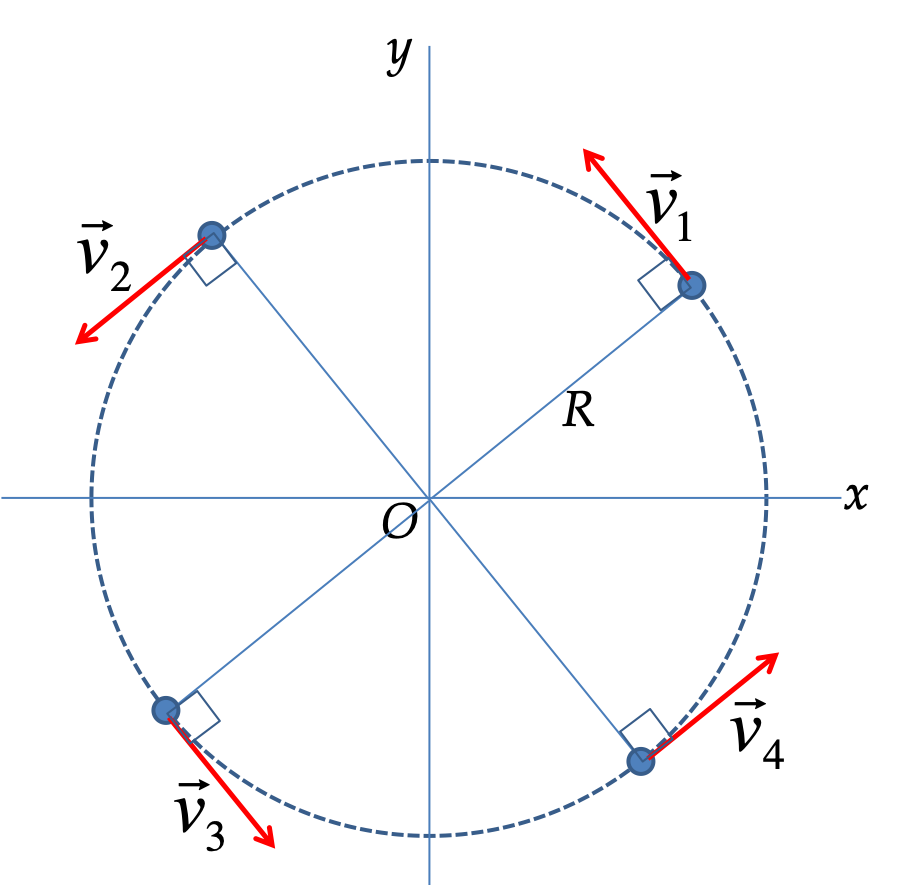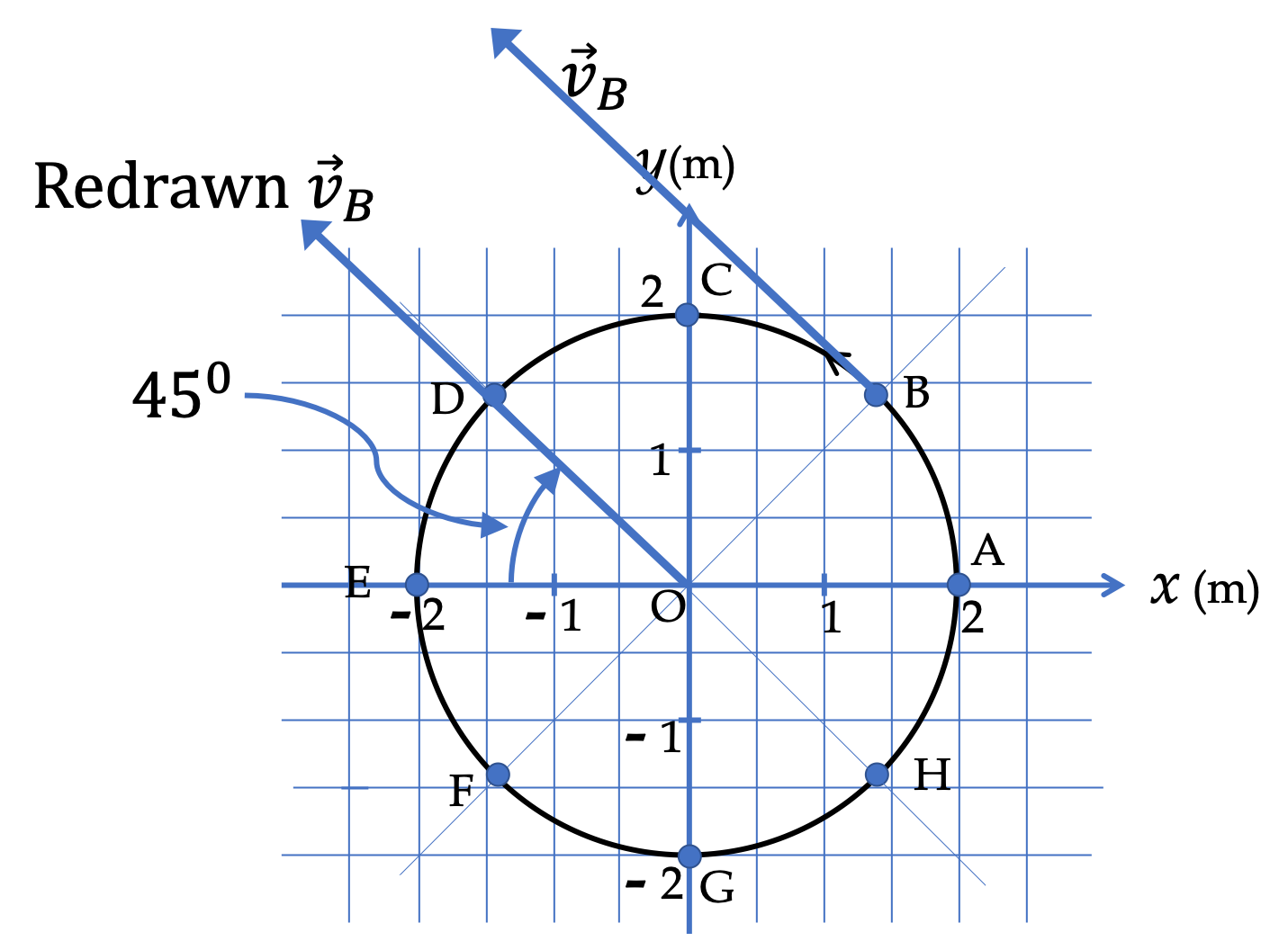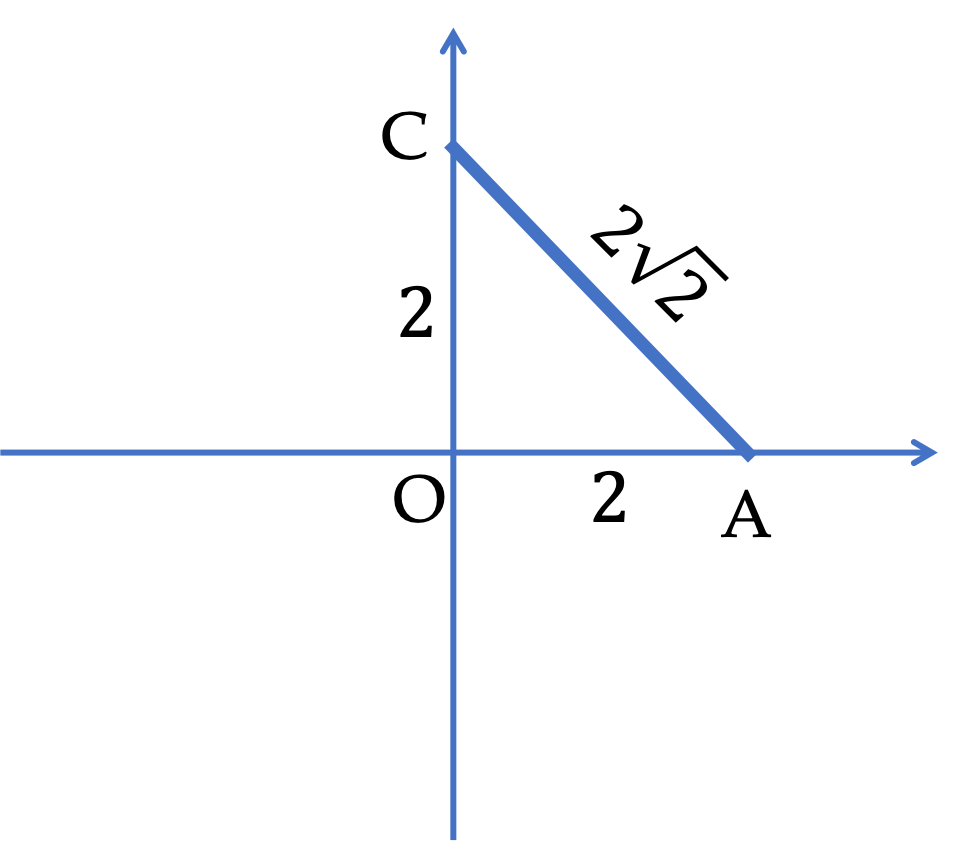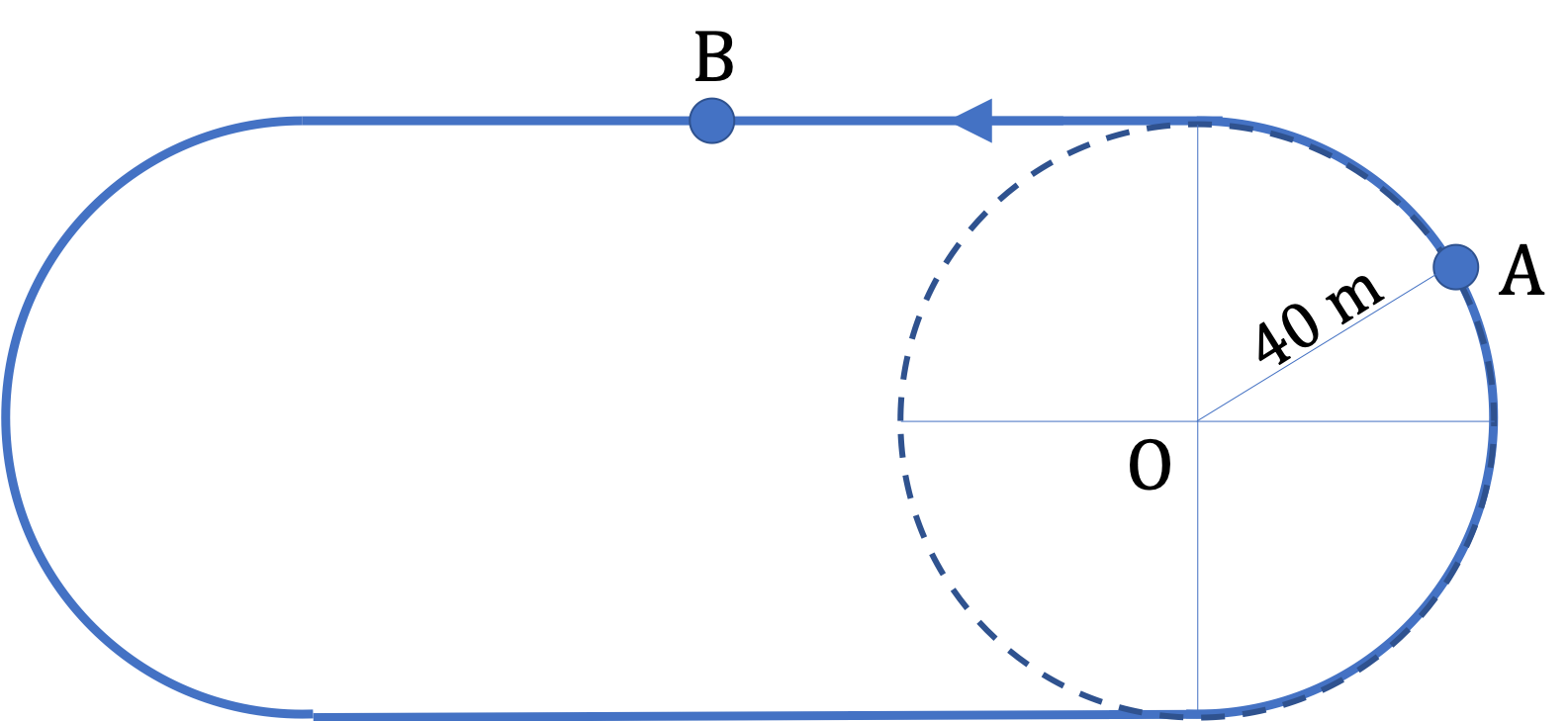## Section5.3Uniform Circular Motion

An object in a uniform circular motion goes around a circular path at a constant speed. Since the direction of motion is changing all the time, constant speed does not imply constant velocity. Hence, a uniform circular motion also has acceleration as we will see below.

Let $R$ denote the radius of the circle and $T$ the time to complete one cycle. That is the object covers a distance of one circumference, $2\pi R\text{,}$ in time $t\text{.}$ Then, average speed $v$ of motion will be

\begin{equation} v = \dfrac{2\pi R}{T}.\tag{5.3.1} \end{equation}

Since in uniform motion speed is constant, speed at any instant on the circle has this same speed.

Now, you would think that requiring speed to be constant in the motion will severely limit application of formulas in thise section. It turns out that, even in places of nonuniform on circular arc, not necessarily motion in a circle, an application of formulas of this section provides a good starting point. A case in point is the application of uniform circular motion formulas to the elliptical motion of Earth around Sun, where motion is neither circular nor uniform.

### Subsection5.3.1Velocity Directions in a Circular Motion

In general, a tangent to the trajectory of motion gives the direction of velocity. In the case of a circle, the tangent is perpendicular to the radial line from the center of the circle. As you can immediately see in Figure 5.3.1, the direction of tangent is different at different points of the circle. Therefore, even though, magnitude of velocity, i.e., speed, is same at all points of a uniform motion, velocity itself changes with time.Figure 5.3.1. Velocity at any point on the circle is in the direction of the tangent to the circle at that point. The lengths of the arrows correspond to the values of the speed. In a constant-speed motion, the lengths of the arrows remain same, but their directions in space changes.

Thus, we can describe velocity vector, $\vec v$ for a uniform circular motion in a circle of radius $R$ and period $T$ as follows.

\begin{align*} \amp \text{Magnitude } = v = \frac{2\pi R}{T},\\ \amp \text{Direction: tangent to the circle. } \end{align*}

A block of steel is tied to a string. By holding the other side of the string, the block is swung in a circle of radius $R=50\text{ cm}\text{.}$ It takes $4.0\text{ s}$ to complete 10 complete cycles. (a) What is the average speed of the block? (b) Is the motion a uniform circular motion?

Hint

(a) Use $d/t\text{.}$

(a) $7.85\text{ m/s}\text{,}$ (b) We do not know if the motion has steady speed at all instants.

Solution 1 (a)

(a) We can get average speed of the block by dividing the net distance traveled by the time. In 10 cycles, the distance covered will be 10 times circumference. Therefore, average speed

\begin{align*} v_\text{s,av} \amp = \dfrac{10\times 2\pi R}{t}\\ \amp = \dfrac{20\times \pi \times 0.5\text{ m}}{4.0\text{ s}} = 7.85\text{ m/s}. \end{align*}
Solution 2 (b)

(b) We do not know if the motion has steady speed at all instants. We only know what happened, on average.

A child in a merry-go-round is moving in a circle of radius $5\text{ m}$ at a constant speed of $2.0\text{ m/s}\text{.}$

(a) What is the distance on the arc the child moves during a $4.0\text{ sec}$ interval?

(b) How much angle in radians the child covers during a $4.0\text{ sec}$ interval?

(c) What is the angular speed of the child?

(d) At $t= 0 \text{,}$ the child was towards East from the center and was moving towards North, what is direction of the child at $t = 100\text{ sec}\text{?}$

Hint

(a) $s=vt$ , (b) $s= R \theta \text{,}$ (c) definition, (d) Find the cumulative angle, and then find the angle within the range 0 to $360^\circ\text{.}$

(a) $8.0\text{ m}\text{,}$ (b) $1.6\text{ rad}\text{,}$(c) $0.4\text{ rad/sec} \text{,}$(d) $131.83^{\circ}$ counterclockwise from positive $x$ axis.

Solution 1 (a)

(a) Since speed is constant, the distance will simply be the product of speed and time.

\begin{equation*} s = v t = 2.0 \times 4.0 = 8.0\text{ m}. \end{equation*}
Solution 2 (b)

(b) The distance $8.0\text{ m}$ on the arc will translate into the angle subtended by $s = R\theta$ formula.

\begin{equation*} \theta = \dfrac{s}{R} = \dfrac{8}{5} = 1.6\text{ rad}. \end{equation*}
Solution 3 (c)

(c) Rotated $1.6\text{ rad}$ in $4.0\text{ sec}\text{.}$ Therefore, average angular speed will be

\begin{equation*} \omega = \dfrac{1.6}{4} = 0.4\text{ rad/sec}. \end{equation*}

We could also get this result by using the formula relating angular velocity to the speed.

\begin{equation*} \omega = \dfrac{v}{R} = \dfrac{2}{5} = 0.4\text{ rad/sec}. \end{equation*}
Solution 4 (d)

(d) The angle at instant $t = 100\text{ sec}$ has to be placed between $0$ and $360^{\circ}$ by dividing the cumulative angle by $360\text{,}$ and throwing away the multiples. Let's first work out the cumulative angle.

\begin{equation*} \theta_\text{cumulative} = 0.4\text{ rad/sec}\times 100\text{ s} \times \frac{180}{\pi}= 2291.83^{\circ}. \end{equation*}

Now, we divide this by $360\text{.}$

\begin{equation*} 2291.83/360 = 6.366194. \end{equation*}

Throwing the integral part and then multiplying the fractional part by 360 we get the counterclockwise angle from the positive $x$ axis.

\begin{equation*} \theta = 0.366194 \times 360 = 131.83^{\circ}. \end{equation*}

### Subsection5.3.2Centripetal Acceleration

Acceleration is the rate of change of velocity. Normally, acceleration either speeds up the motion or slows it down. But, here neither speed up or slowing down of the motion occurs, but instead, the role of acceleration is to change the direction of velocity.

To appreciate change in velocity during a small interval, we can draw velocity vectors for two nearby instances and find their difference vector as shown in Figure 5.3.4. The direction of acceleration will be in the direction of the vector $\Delta \vec v\text{,}$ which is pointed towards the center of the circle.

The diagram in Figure 5.3.4 gives us direction of acceleration to be towards the center of the circle. A direction towards the center is also called centripetal; hence, this acceleration is also called centripetal acceleration. To get the magnitude from this diagram, you can carefully draw arrows to scale and estimate magnitude of $\Delta \vec v\text{.}$ Then, you would divide this magnitude by $\Delta t\text{.}$ Instead of doing that, an analytic calculation, which will be presented when we discuss circular motion in polar coordinates, shows us that the magnitude of centripetal acceleration $a_c$ has the formula.

\begin{equation} a_c = \frac{v^2}{R}.\tag{5.3.2} \end{equation}

Note that, although the magnitude of the centripetal acceleration, $v^2/R\text{,}$ does not change since $v$ and $R$ are constant, the direction of the acceleration in space changes all the time. Hence, acceleration (vector) is not constant in a uniform circular motion as illustrated in Figure 5.3.5.

ALERT:Formulas of constant acceleration you have learned in earlier chapters are not applicable for uniform motion!

A child in a merry-go-round is moving in a circle of radius $5\text{ m}$ at a constant speed of $2.0\text{ m/s}\text{.}$

(a) What is the acceleration of the child at the instant the child is in the direction of East from the center?

(b) What is the acceleration of the child at the instant the child is in the direction of North from the center?

Hint

Be mindful of direction!

(a) $0.8 \text{ m/s}^2$ West, (b) $0.8 \text{ m/s}^2$ South

Solution 1 (a)

The magnitude of acceleration will be constant, but its direction will be different depending on where in the circle the child happens to be.

\begin{equation*} \text{Magnitude, }a = \dfrac{v^2}{R} = \dfrac{2.0^2}{5} = 0.8\text{ m/s}^2. \end{equation*}

(a) The acceleration at this instant has magnitude $0.8\text{ m/s}^2$ towards West, where the center is from the child's position.

Solution 2 (b)

(b) The acceleration at this instant has magnitude $0.8\text{ m/s}^2$ towards South, where the center is from the child's position.

Figure 5.3.9 shows a particle moving in a circle at a constant speed with the positions marked every second. The circular path has been placed on a grid with the distances as marked.

(a) Express the position of the particle at A, B, C, D, E, F, G, and H in the polar coordinates, i.e., read off the distances $r$ and angles $\theta$ in radian going counterclockwise from the positive $x$ axis.

(b) Express the position of the particle at A, B, C, D, E, F, G, and H in the Cartesian coordinate form, i.e., give $(x,y)$ of each point.

(c) What are the speed and angular speed of the particle at these points?

(d) What are the velocity of the particle at these points?

(e) What are the centripetal acceleration of the particle at these points?

(f) What are the tangential acceleration of the particle at these points?

(g) What are the acceleration of the particle at these points?

(h) Your friend claims that the magnitude of the average velocity between A and C is greater than that between A and E. Is he right? Compute them and compare.

Hint

(a) same $r \text{,}$ (b) exploit symmetry since angles are all $\pm45^{\circ}$ , (c) find average since constant, (d) think direction also, (e) formula and think direction, (f) zero, (g) think definition, (h) direct distance will be helpful.

See solutions.

Solution 1 (a), (b)

(a) First note that $r = 5\text{ m}$ for every point on the circle. The angles in radian are: $\theta_A = 0\text{,}$ $\theta_B = \dfrac{\pi}{4}\text{ rad}\text{,}$ $\theta_C = \dfrac{\pi}{2}\text{ rad}\text{,}$ $\theta_D = \dfrac{3\pi}{4}\text{ rad}\text{,}$ $\theta_E = \pi\text{ rad}\text{,}$ $\theta_F = \dfrac{5\pi}{4}\text{ rad}\text{,}$ $\theta_G = \dfrac{3\pi}{2}\text{ rad}\text{,}$ $\theta_H = \dfrac{7\pi}{4}\text{ rad}\text{.}$

(b) For A, C, E, and G, we don't need any work.

\begin{align*} \amp (x_A = 5\text{ m},\ y_A = 0 ) \\ \amp (x_C = 0,\ y_C = 5\text{ m} ) \\ \amp (x_E = -5\text{ m},\ y_E = 0 ) \\ \amp (x_G = 0,\ y_G = -5\text{ m} ) \end{align*}

For B, D, F, and G, we just need to work out the $x$ of B since all these are at $45^{\circ}$ form either positive or negative $x$ axis. Then use it to write $(x,y)$ of B, D, F, and H.

\begin{equation*} x_B = 2\, \cos\, 45^{\circ} = 1.414\text{ m}. \end{equation*}

Therefore,

\begin{align*} \amp (x_B = 1.414\text{ m},\ y_B = 1.414\text{ m} ) \\ \amp (x_D = -1.414\text{ m},\ y_D = 1.414\text{ m} ) \\ \amp (x_F = -1.414\text{ m},\ y_F = -1.414\text{ m} ) \\ \amp (x_H = 1.414\text{ m},\ y_H = -1.414\text{ m} ) \end{align*}
Solution 2 (c), (d)

(c) Since the speed is constant, we need to work out just the average speed, which would be just an arc length between any two points divided by time it took. Between A and B, the arc length is

\begin{equation*} s_{AB} = R\theta = 2\times\dfrac{\pi}{4} = 1.57\text{ m}. \end{equation*}

This takes $1\text{ sec}\text{.}$ Therefore, the speed is

\begin{equation*} v_s = \dfrac{1.57\text{ m}}{1\text{ s}} = 1.57\text{ m/s}. \end{equation*}

The angle between A and B was $\pi/4\text{ rad}\text{.}$ Therefore, the angular speed is

(d) Since speed is constant, the magnitude of velocity is cinstant also.

\begin{equation*} \text{Magnitude: } 1.57\text{ m/s}. \end{equation*}

But, the direction of velocity at differnet points are actually different - that makes velocity different at different points here.

A. The velocity at A is pointed towards positive $y$ axis.

B. At point B, the velocity has magnitude $1.57\text{ m/s}\text{,}$ same as speed there, and the direction is towards $45^{\circ}$ clockwise from the negative $x$ axis as shown in the figure below. Note how I drew the valeocity as tangent to the circle at point B and then redrew a vector parallel to the velocity vector but the tail at the origin.C. The velocity at C is pointed towards negative $x$ axis.

D. The velocity at D is pointed $45^{\circ}$ counterclockwise from the negative $x$ axis

E. The velocity at E is pointed towards negative $y$ axis.

F. The velocity at F is pointed $45^{\circ}$ clockwise from the positive $x$ axis

G. The velocity at G is pointed towards positive $x$ axis.

H. The velocity at H is pointed $45^{\circ}$ counterclockwise from the positive $x$ axis

Solution 3 (e), (f), (g)

(e) The centripetal acceleration at all points have the same magnitude since speed is constant.

\begin{equation*} \text{Magnitude } = \dfrac{v^2}{R} = \dfrac{1.57^2}{2} = 0.785\text{ m/s}^2. \end{equation*}

The direction at various points are pointed towards the center of the circle. Thus, at A, the direction is towards the negative $x$ axis. At B, it is $45^{\circ}$ counterclockwise to the negative $x$ axis, at C, it is pointed towards the negative $y$ axis, at D, it is towards $45^{\circ}$ clockwise from the positive $x$ axis, at E, it is towards the positive $x$ axis, at F, it is towards $45^{\circ}$ counterclockwise from the positive $x \text{,}$ at G it is towards the positive $y$ axis, and at H it is towards $45^{\circ}$ clockwise to the negative $x$ axis.

(f) The tangential acceleration is zero since speed is constant.

(g) Acceleration is the vector sum of centripetal and tangential accelerations. But since the tangential acceleration is zero, acceleration is same as the centripetal acceleration.

Solution 4 (h)

(h) Your friend is correct. Lets work out the magnitudes of the average velocity by working out the direct distances and dividing by the corresponding times.

Between A and C, the direct distance can be obtained by the triangle shown.

\begin{equation*} d_{\text{AC}} = 2\sqrt{2} = 2.83\text{ m}. \end{equation*}

This takes $2\text{ sec}\text{.}$ Therefore, the magnitude of the averafge velocity is

\begin{equation*} v_{\text{AC}} = \dfrac{2.83}{2} = 1.42\text{ m/s}. \end{equation*}Between A and E, the direct distance is $4 \text{ m}$ and the time is $4 \text{ s}\text{.}$ Therefore, the magnitude of the average velocity is

\begin{equation*} v_{AE} = \dfrac{4 \text{ m}}{4 \text{ s}} = 1.0\text{ m/s}. \end{equation*}

The calculations bear out the correctness of the claim by your friend.

The figure shows an oval track with two ends arcs of circles of radius $40 \text{ m}\text{.}$ You are running on the track at a constant speed of $2.0 \text{ m/s} \text{.}$

Find the magnitude and direction of accelerations at the two points marked (a) A and (b) B.Hint

(a) centripetal, (b) tricky.

(a) $0.1\text{ m/s}^2$ in the directoin from A to O. (b) Zero.

Solution 1 (a)

(a) The acceleration here is the centripetal acceleration towards O, the center of the circular arc with magnitude

\begin{equation*} a_A = \dfrac{v^2}{R} = \dfrac{2^2}{40} = 0.1\text{ m/s}^2. \end{equation*}
Solution 2 (b)

(b) At the instant you are at B, not only your speed is constant, but your direction is also constant. Hence, your velocity is constant. Therefore, the acceleration is zero here.

A particle moves in a circle of radius $20\ \text{cm}$ and centered at $(x = 1\ \text{m}, y=0)$ in the $xy$-plane with a constant angular speed of $50 \text{ rad/sec}\text{.}$ At $t=0\text{,}$ the particle is at $(x = 1.2\ \text{m}, y=0)\text{.}$

(a) Give an expression of the position of the particle at an arbitrary time $t\text{?}$ (b) For the particle moving in a counterclockwise sense, find velocity at $t=0\text{?}$

Hint

(a) Use center of circle and redius to get $x$ and $y\text{.}$ (b) Take derivative.

(a) $x = 1 + 0.2 \cos(50 t),\ \ y = 0.2 \sin(50 t)\text{,}$ (b)$10\text{ m/s}\ \hat j\text{.}$

Solution 1 (a)

(a) From the fact that the motion is circular with center at $(1\text{ m},0)$ and radius $R=0.2\text{ m}\text{,}$ we can immediately write $x$ and $y$ coordinates

\begin{equation*} x = 1 + 0.2\; \cos(\omega t),\ \ y = 0.2\; \sin(\omega t), \end{equation*}

where $\omega = 50 \text{ rad/sec}\text{.}$

Solution 2 (b)

(b) Taking derivatives we get the components, which we evaluate at $t=0\text{.}$

\begin{align*} v_x \amp = -0.2\;\omega\; \sin(\omega t) = 0,\\ v_y \amp = 0.2\;\omega\; \cos(\omega t) = 10\text{ m/s}. \end{align*}

The velocity has magnitude $10\text{ m/s}$ and directed towards positive $x$ axis.

The position of a particle moving in the $xy$-plane is given by $\vec r(t) = a \cos(\pi t) \hat i + (b + a \sin(\pi t)) \hat j\text{,}$ where $t$ is in $\text{sec}$ and $a$ in $\text{cm}\text{.}$ (a) Show that the motion is a circular motion, and find the value of the radius of the circle, and Cartesian coordinates of the center of the circle. (b) Find an expression for the velocity of the particle at an arbitrary instant $t\text{.}$ (c) Find an expression for the angular speed of the particle at an arbitrary time $t\text{.}$ (d) Find an expression of the acceleration of the particle at an arbitrary instant $t\text{.}$

Hint

(a) Look at components of the position vector. (b) Take drivative. (c) Use relation of angular speed and speed. (d) Take derivative.

(a) $x^2 + (y - b)^2 = a^2\text{,}$ (b) $v = \pi a \text{,}$ $\hat u_v = -\hat i\, \sin(\pi t)+ \hat j\, \cos(\pi t)\text{,}$ (c) $\pi\text{,}$ (d) $- \pi^2 a \left( \hat i \cos(\pi t) + \hat j\, \sin(\pi t) \right)\text{.}$

Solution 1 (a)

(a) From the given position vector we have $x$ and $y$ of the particle to be

\begin{equation*} x(t) = a \cos(\pi t),\ \ y(t) = b + a \sin(\pi t). \end{equation*}

From this we see that

\begin{equation*} x^2 + (y - b)^2 = a^2. \end{equation*}

This is equation of a circle with radius $a$ and center at $(0,b)\text{.}$

Solution 2 (b)

(b) From $x$ and $y\text{,}$ we get $v_x$ and $v_y$ as usual.

\begin{align*} v_x \amp \dfrac{dx}{dt} = - \pi\, a\, \sin(\pi t),\\ v_y \amp \dfrac{dy}{dt} = \pi\, a\, \cos(\pi t), \end{align*}

This gives magnitude

\begin{equation*} v = \pi a, \end{equation*}

and direction in the direction of the unit vector

\begin{equation*} \hat u_v = -\hat i\, \sin(\pi t)+ \hat j\, \cos(\pi t), \end{equation*}

which shows explicitly that direction of velocity is changing with time.

Solution 3 (c)

(c) Angular speed will be equal to the magnitude of velocity divided by radius of the circle. This gives

\begin{equation*} \omega = \dfrac{\pi a}{a} = \pi. \end{equation*}
Solution 4 (d)

(d) Acceleration will be obtained by taking derivative of the velocity.

\begin{equation*} \vec a = - \pi^2 a \left( \hat i \cos(\pi t) + \hat j\, \sin(\pi t) \right). \end{equation*}